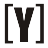How to Convert Decimal to Hexadecimal: insert number in Decimal box and press convert button to get result in Hexadecimal.

10
16
16
2

Little endian

Big endian

Decimal to hex calculation steps

Divide by the base 16 to get the digits from the remainders:

Division
by 16
Quotient

Remainder

(Digit)
Digit #

Hex to Decimal Converter

Hexadecimal is a number system that uses base 16 instead of base 10 like the decimal system. In hexadecimal, the numbers are represented using 16 symbols, including 0-9 and A-F. Each digit in a hexadecimal number represents a value of a power of 16, starting from the rightmost digit.

## How to convert Decimal to Hexadecimal

To convert a decimal number to hexadecimal, you can use the following steps:

1. Divide the decimal number by 16.
2. Write down the integer quotient and remainder.
3. Convert the remainder to a hexadecimal digit (0-9, A-F).
4. If the quotient is greater than 0, repeat steps 1 to 3 using the quotient as the new decimal number.
5. Write down the resulting hexadecimal digits in reverse order.
You can use this formula to convert any decimal number to hexadecimal by following these steps:
2. Divide the decimal number by 16, and write down the quotient and remainder.
3. Convert the remainder to a hexadecimal digit using the following table:

0 0
1 1
2 2
3 3
4 4
5 5
6 6
7 7
8 8
9 9
10 A
11 B
12 C
13 D
14 E
15 F

4. If the quotient is greater than 0, repeat steps 2 to 4 using the quotient as the new decimal number.
5. Write down the resulting hexadecimal digits in reverse order.

This formula works for converting any positive decimal number to its equivalent hexadecimal representation.

Examples:

1. Divide the decimal number by 16.
4331 ÷ 16 = 270 remainder 11
2. Convert the remainder (11) to a hexadecimal digit. Since 11 is equivalent to the hexadecimal digit B, write down B as the least significant digit of the resulting hexadecimal number.
3. Divide the quotient (270) by 16.
270 ÷ 16 = 16 remainder 14
4. Convert the remainder (14) to a hexadecimal digit. Since 14 is equivalent to the hexadecimal digit E, write down E as the next digit of the resulting hexadecimal number.
5. Divide the quotient (16) by 16.
16 ÷ 16 = 1 remainder 0
6. Convert the remainder (0) to a hexadecimal digit. Since 0 is equivalent to the hexadecimal digit 0, write down 0 as the next digit of the resulting hexadecimal number.
7. Since the quotient (1) is less than 16, write it down as the most significant digit of the resulting hexadecimal number.
8. Write down the resulting hexadecimal digits in order, from most significant to least significant.
The resulting hexadecimal number is 10E3.

Therefore, the decimal number 4331 is equivalent to the hexadecimal number 10E3 in base 16.

### Decimal to Hex Conversion Table

0 0
1 1
2 2
3 3
4 4
5 5
6 6
7 7
8 8
9 9
10 A
11 B
12 C
13 D
14 E
15 F
16 10
17 11
18 12
19 13
20 14
21 15
22 16
23 17
24 18
25 19
26 1A
27 1B
28 1C
29 1D
30 1E
31 1F
32 20
254 FE
255 FFYoosFuhl.com Would you like to receive notifications on latest updates? No Yes